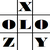anything in Excel...

It's Excel-o-lozy...

### Excel Formula to convert Date from to valid date format like YYYYMMDD to DDMMYYYY - an update

www.Excelolozy.com - "Formula Fix" was created to Convert YYYYMMDD to DDMMYYYY i.e. the Date from Raw text format to recognisable and valid Date format.

Now it works on a single digit year format too.

Following was the Formula (read here full post):

formula is "ConvertDate"

=ConvertDate(Ref,OldFormat)

This tool used to work with a two digit year like "98" means 2008.

As per need of an Excelolozy user we have upgraded it to Single Digit year format too.

i.e. it now converts  "8" as "2008".

The formula "ConvertDate" is available within tool named "FormulaFix".

"FormulaFix" is a "Formula (fx) Fixing Tool" that provides various formulas that don't exist in Excel.

Examples of formula "ConvertDate" are as follows :

 Raw Date Old Format Formula Converted Date result Remarks 20121013 if omitted =convertdate(A2,) Saturday, October 13, 2012 YYYYMMDD is taken by default if omitted in the formula. 13102012 DDMMYYYY =convertdate(A3,B3) Saturday, October 13, 2012 Any type of OLD FORMAT can be converted to any type of NEW FORMAT. 322012 DMYYYY =convertdate(A4,B4) Friday, February 03, 2012 2313 DMYY =convertdate(A5,B5) Saturday, March 02, 2013 2032013 DMMYYYY =convertdate(A6,B6) Saturday, March 02, 2013 2013 03 2 YYYY MM D =convertdate(A7,B7) Saturday, March 02, 2013 20130302 YYYYMMDD =convertdate(A8,B8) Saturday, March 02, 2013 03-02-2013 MM-DD-YYYY =convertdate(A9,B9) Saturday, March 02, 2013 Any character of OLD FORMAT can be omitted by placing any character in NEW FORMAT. 13aaa3aaa02 YYxxxMxxxDD =convertdate(A10,B10) Saturday, March 02, 2013 13bbb3bbb02 YYxxxMxxxDD =convertdate(A11,B11) Saturday, March 02, 2013

To see practical application of above table do as follows :